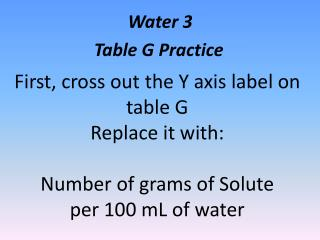Download PresentationWater 3 Table G Practice

# Water 3 Table G Practice - PowerPoint PPT PresentationDownload Presentation## Water 3 Table G Practice

- - - - - - - - - - - - - - - - - - - - - - - - - - - E N D - - - - - - - - - - - - - - - - - - - - - - - - - - -
##### Presentation Transcript

1. First, cross out the Y axis label on table G Replace it with: Number of grams of Soluteper 100 mL of water Water 3 Table G Practice

2. Are these solutions SATURATED or UNSATURATED? If they are unsaturated, how many more grams of solute would fit into the solution?

3. three

4. four

5. five

6. six

7. seven

8. eight

9. nine

10. ten

11. Using Table G • 1. How many grams of potassium nitrate saturates 100 mL water at 60°C? • 2. Could you dissolve 130 g potassium nitrate into 100 mL of this water? • 3. Could you dissolve 40 g NaCl into 100 mL water at 70 °C? • What’s the saturation level for sodium chloride in 100 mL of 70 °C aqueous solution? • THINK hard, look hard, write down 4 answers

12. 1. How many grams of potassium nitrate saturates 100 mL water at 60°C?About 107 grams • 2. Could you dissolve 130 g potassium nitrate into 100 mL of this water?No, it’s saturated by 107 grams • 3. Could you dissolve 40 g NaCl into 100 mL water at 70 °C?No, it’s saturated at 38 grams • What’s the saturation level for sodium chloride in 100 mL of 70 °C aqueous solution?About 38 grams

13. Assume you have a saturated 100 mL aqueous solution of KClO3 at 50°C. What happens if you cool it to 45°C? Cool to forty Start at fifty

14. Assume you have a saturated 100 mL aqueous solution of KClO3 at 50°C. What happens if you cool it to 45°C? Cool to forty Only 15 grams KClO3 fits at this cooler temperature Start at fifty 22 grams of KClO3 fits into solution. 7 grams precipitates out of solution.

15. Easy question first, then another, then a harder one. Assume you have a 100 mL saturated solution of NaNO3 at 20°C, and you warm it up to 50°C. How much more solute will be able to dissolve?How many grams of ammonia will dissolve into 100 mL water at 90°C? How many grams of ammonia will dissolve into just 40 mL water at the same temperature?

16. Assume you have a 100 mL saturated solution of NaNO3 at 20°C, and you warm it up to 50°C. How much more solute will be able to dissolve?At 50 degrees about 115 g At 20 degrees about 88 g 27 more grams could dissolve into the warmer solutionHow many grams of ammonia will dissolve into 100 mL water at 90°C? How many grams of ammonia will dissolve into just 40 mL water at the same temperature?

17. Assume you have a 100 mL saturated solution of NaNO3 at 20°C, and you warm it up to 50°C. How much more solute will be able to dissolve?At 50 degrees about 115 g At 20 degrees about 88 g 27 more grams could dissolve into the warmer solutionHow many grams of ammonia will dissolve into 100 mL water at 90°C?10 grams How many grams of ammonia will dissolve into just 40 mL water at the same temperature? Ammoniawater 10 g100 mL X g40 mL 90°C = 100 x = 400 X = 4 g ammonia

18. How many grams NaCl dissolves into 43 mL water at 90°C? How many grams sodium nitrate saturates 1000 mL water at 10°C?

19. How many grams NaCl dissolves into 43 mL water at 90°C? NaClwater 40g100 mL X g43 mL 90°C 100 x = 1720 X = 17.2 grams = 17 grams with 2 SF

20. How many grams sodium nitrate saturates 1000 mL water at 10°C? 80g100 mL X g1000 mL NaNO3water 10°C 100 x = 80000 X = 800 grams

21. How many grams KCl saturates 875 mL water at 10°C? How many grams sodium nitrate dissolve into 1420 mL water at 50°C? How many grams ammonium chloride dissolve into 25 mL water at 70 °C?

22. How many grams KCl saturates 875 mL water at 10°C? KClwater 30 g100 mL X g875 mL 10°C 100 X = 26250 X = 262.5 = 263 g 3 SF

23. How many grams sodium nitrate dissolve into 1420 mL water at 50°C? NaNO3water 115 g100 mL X g1420 mL 50°C 100 X = 163,300 X = 1633 = 1630 g 3 SF

24. How many grams ammonium chloride dissolve into 25.0 mL water at 70 °C? 15.5 = 16 grams with 2 SF NH4Clwater 62 g100 mL X g25.0 mL 70°C 100 X = 1550 X = 15.5 g ammonium chloride